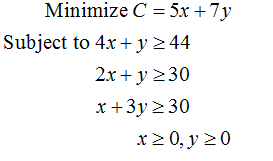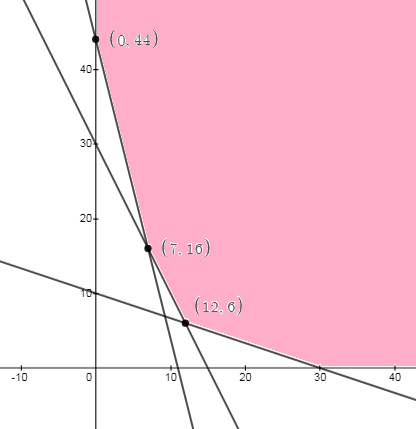# Solve the linear programming problem by the method of corners.MinimizeC = 5x + 7y    subject to  4x + y ≥44 2x + y ≥30 x + 3y ≥30 x ≥ 0, y ≥ 0  The minimum is C = (x, y) =   .

Question
12 views

Solve the linear programming problem by the method of corners.

 Minimize C = 5x + 7y subject to 4x + y ≥ 44 2x + y ≥ 30 x + 3y ≥ 30 x ≥ 0, y ≥ 0

The minimum is C

(x, y) =

.
check_circle

Step 1

Given minimization problem is,Step 2

Common region is,...

### Want to see the full answer?

See Solution

#### Want to see this answer and more?

Solutions are written by subject experts who are available 24/7. Questions are typically answered within 1 hour.*

See Solution
*Response times may vary by subject and question.
Tagged in